# Neural networks 1 Neural networks Neural networks are

• Slides: 35Neural networks 1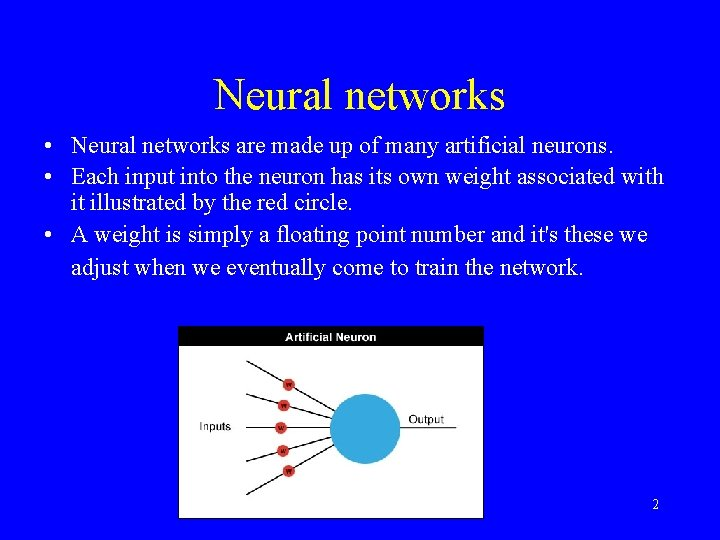Neural networks • Neural networks are made up of many artificial neurons. • Each input into the neuron has its own weight associated with it illustrated by the red circle. • A weight is simply a floating point number and it's these we adjust when we eventually come to train the network. 2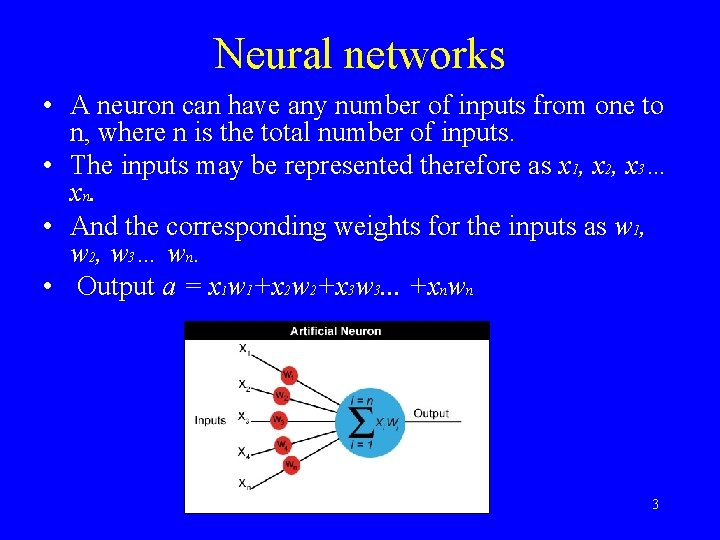Neural networks • A neuron can have any number of inputs from one to n, where n is the total number of inputs. • The inputs may be represented therefore as x 1, x 2, x 3… xn. • And the corresponding weights for the inputs as w 1, w 2, w 3… wn. • Output a = x 1 w 1+x 2 w 2+x 3 w 3. . . +xnwn 3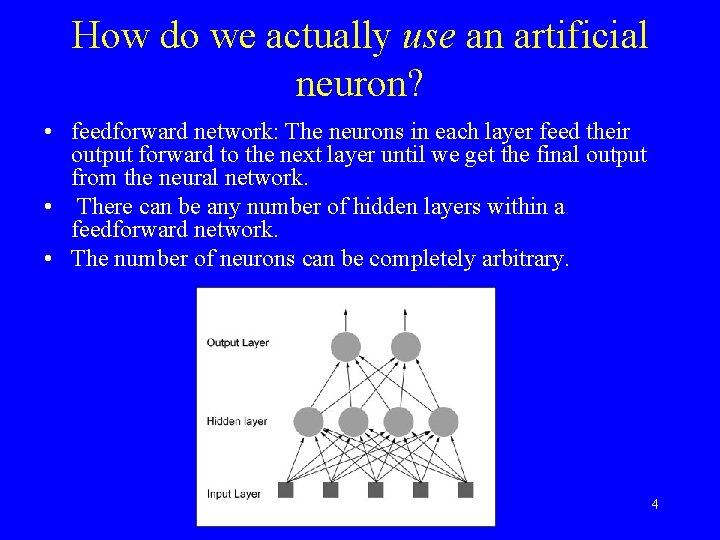How do we actually use an artificial neuron? • feedforward network: The neurons in each layer feed their output forward to the next layer until we get the final output from the neural network. • There can be any number of hidden layers within a feedforward network. • The number of neurons can be completely arbitrary. 4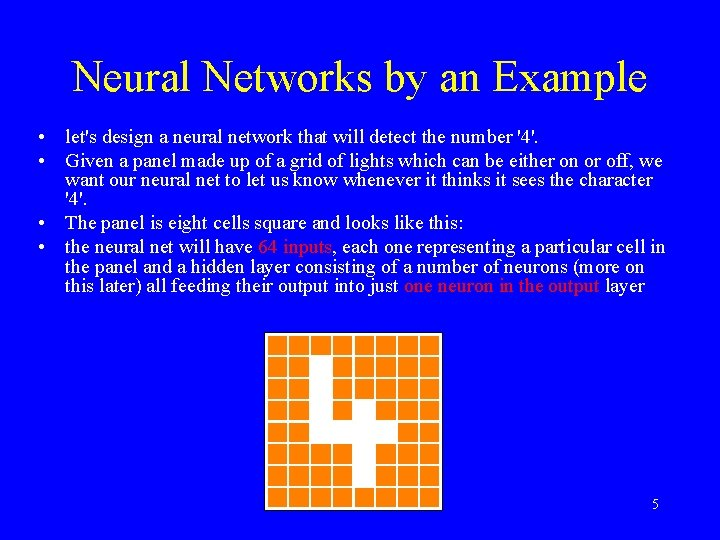Neural Networks by an Example • let's design a neural network that will detect the number '4'. • Given a panel made up of a grid of lights which can be either on or off, we want our neural net to let us know whenever it thinks it sees the character '4'. • The panel is eight cells square and looks like this: • the neural net will have 64 inputs, each one representing a particular cell in the panel and a hidden layer consisting of a number of neurons (more on this later) all feeding their output into just one neuron in the output layer 5Neural Networks by an Example • initialize the neural net with random weights • feed it a series of inputs which represent, in this example, the different panel configurations • For each configuration we check to see what its output is and adjust the weights accordingly so that whenever it sees something looking like a number 4 it outputs a 1 and for everything else it outputs a zero. • More: http: //www. doc. ic. ac. uk/~nd/surprise_96/journal/vol 4/cs 11/rep ort. html 6Multi-Layer Perceptron (MLP) 7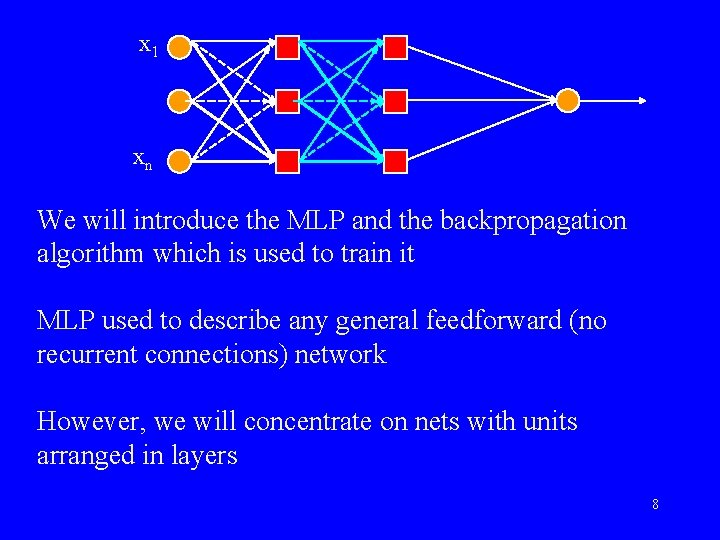x 1 xn We will introduce the MLP and the backpropagation algorithm which is used to train it MLP used to describe any general feedforward (no recurrent connections) network However, we will concentrate on nets with units arranged in layers 8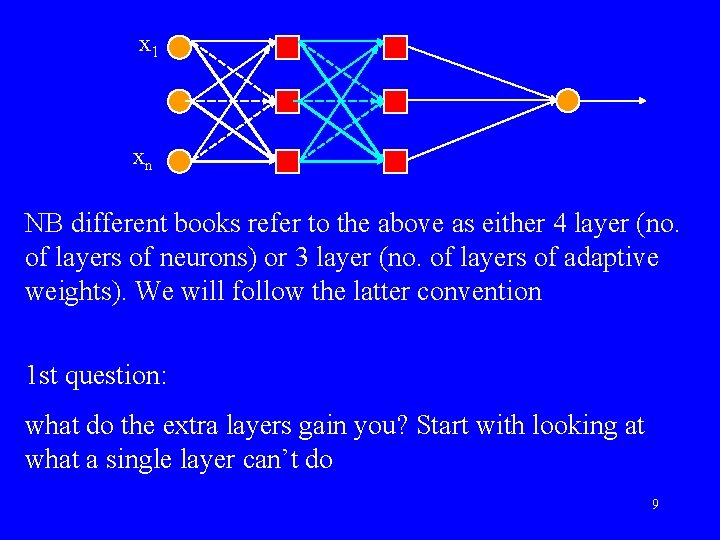x 1 xn NB different books refer to the above as either 4 layer (no. of layers of neurons) or 3 layer (no. of layers of adaptive weights). We will follow the latter convention 1 st question: what do the extra layers gain you? Start with looking at what a single layer can’t do 9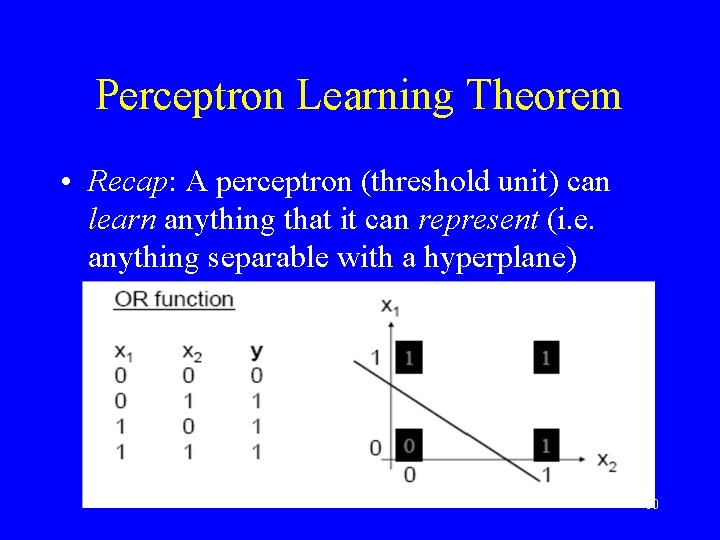Perceptron Learning Theorem • Recap: A perceptron (threshold unit) can learn anything that it can represent (i. e. anything separable with a hyperplane) 10The Exclusive OR problem A Perceptron cannot represent Exclusive OR since it is not linearly separable. 1112Minsky & Papert (1969) offered solution to XOR problem by combining perceptron unit responses using a second layer of Units. Piecewise linear classification using an MLP with threshold (perceptron) units +1 1 3 2 +1 13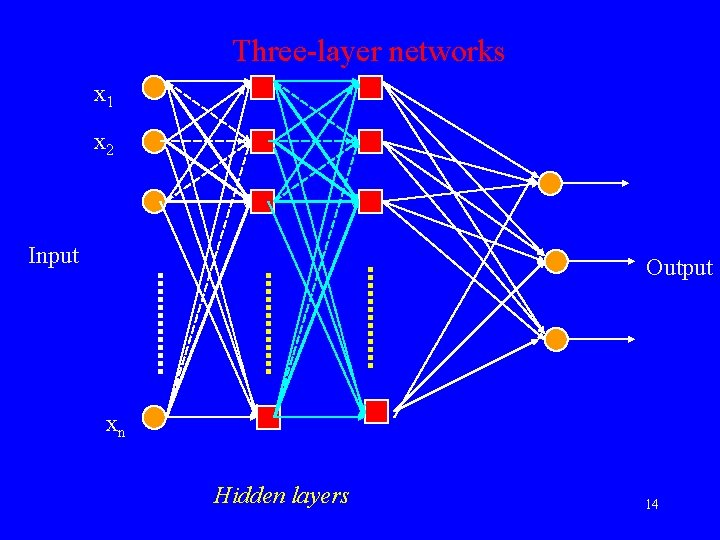Three-layer networks x 1 x 2 Input Output xn Hidden layers 14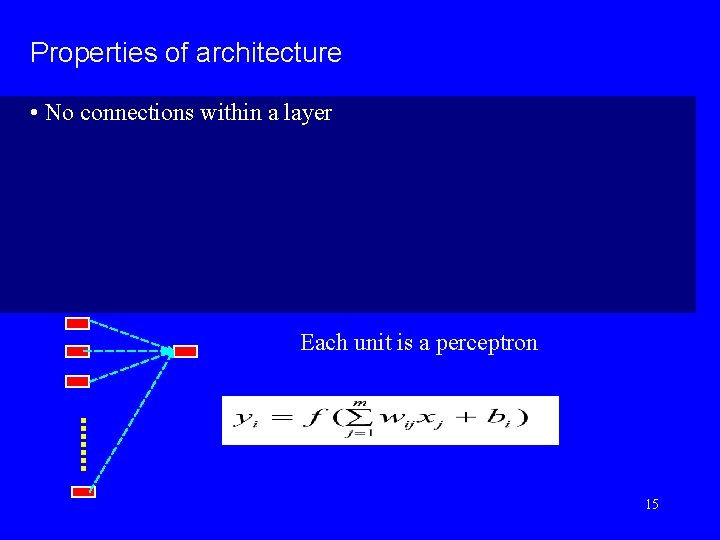Properties of architecture • No connections within a layer Each unit is a perceptron 15Properties of architecture • No connections within a layer • No direct connections between input and output layers • Each unit is a perceptron 16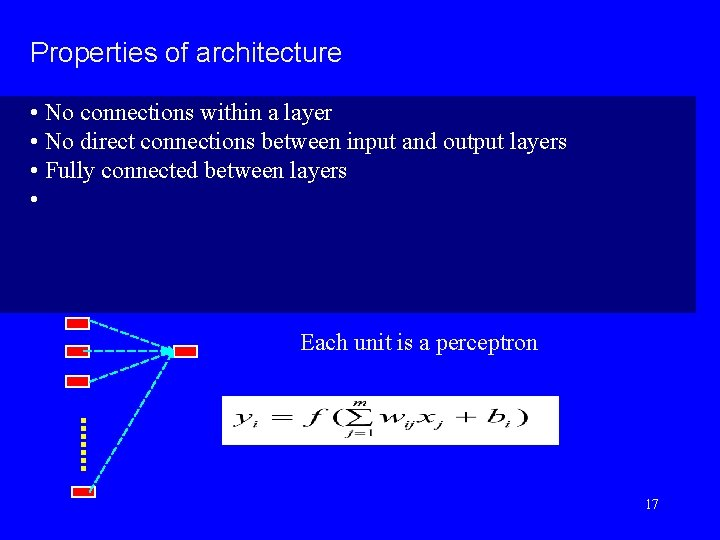Properties of architecture • No connections within a layer • No direct connections between input and output layers • Fully connected between layers • Each unit is a perceptron 17Properties of architecture • No connections within a layer • No direct connections between input and output layers • Fully connected between layers • Often more than 3 layers • Number of output units need not equal number of input units • Number of hidden units per layer can be more or less than input or output units Each unit is a perceptron Often include bias as an extra weight 18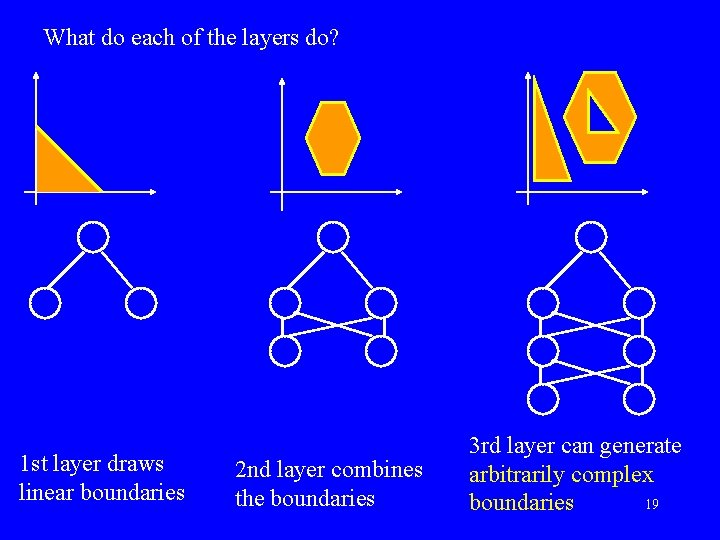What do each of the layers do? 1 st layer draws linear boundaries 2 nd layer combines the boundaries 3 rd layer can generate arbitrarily complex 19 boundariesBackpropagation learning algorithm ‘BP’ Solution to credit assignment problem in MLP. Rumelhart, Hinton and Williams (1986) (though actually invented earlier in a Ph. D thesis relating to economics) BP has two phases: Forward pass phase: computes ‘functional signal’, feed forward propagation of input pattern signals through network Backward pass phase: computes ‘error signal’, propagates the error backwards through network starting at output units (where the error is the difference between actual and desired output values) 20Conceptually: Forward Activity Backward Error 21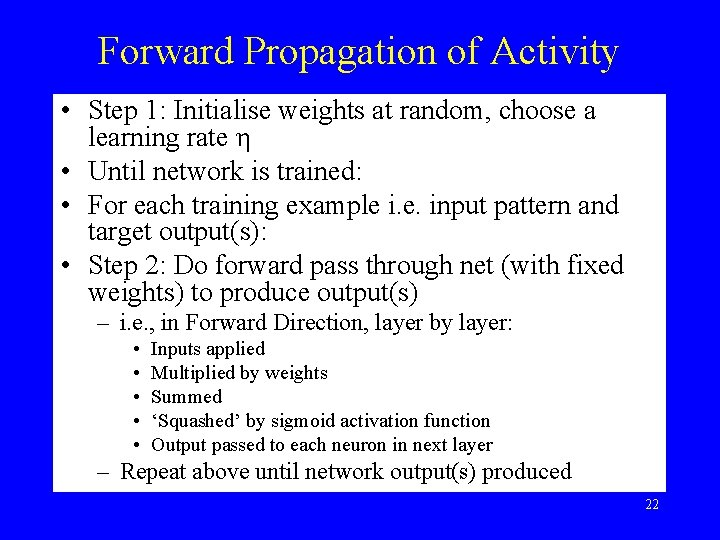Forward Propagation of Activity • Step 1: Initialise weights at random, choose a learning rate η • Until network is trained: • For each training example i. e. input pattern and target output(s): • Step 2: Do forward pass through net (with fixed weights) to produce output(s) – i. e. , in Forward Direction, layer by layer: • • • Inputs applied Multiplied by weights Summed ‘Squashed’ by sigmoid activation function Output passed to each neuron in next layer – Repeat above until network output(s) produced 22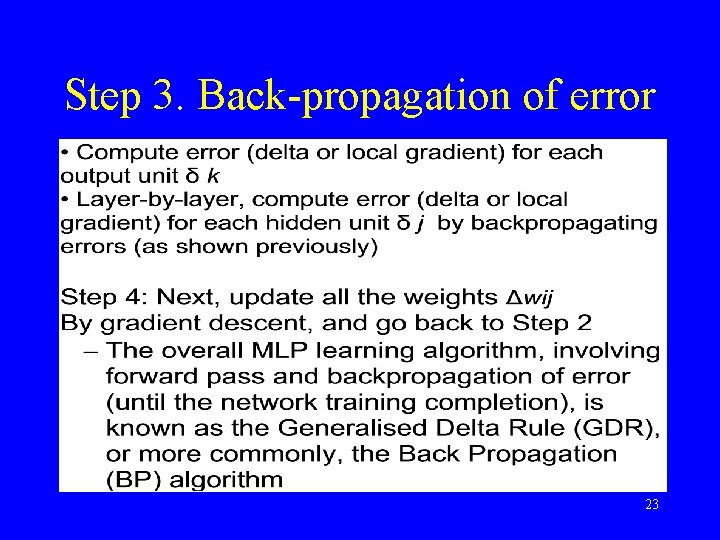Step 3. Back-propagation of error 23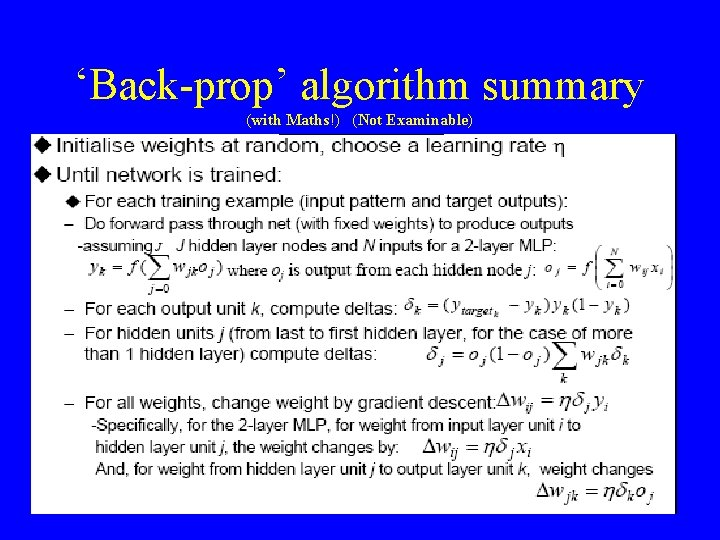‘Back-prop’ algorithm summary (with Maths!) (Not Examinable) 24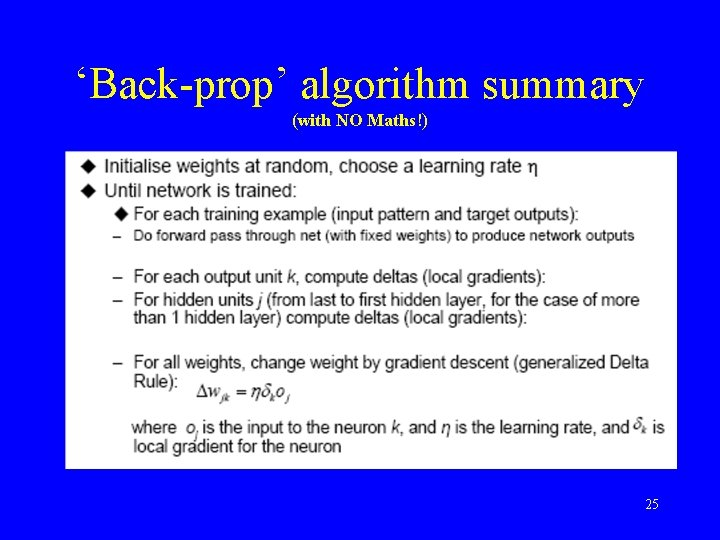‘Back-prop’ algorithm summary (with NO Maths!) 25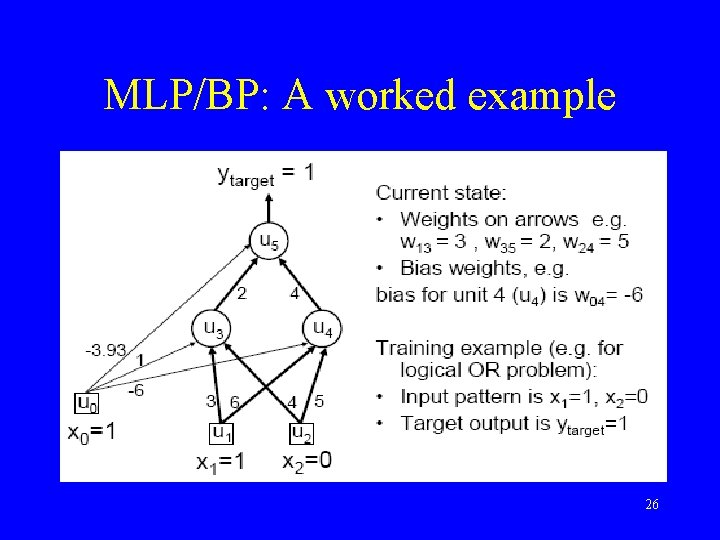MLP/BP: A worked example 26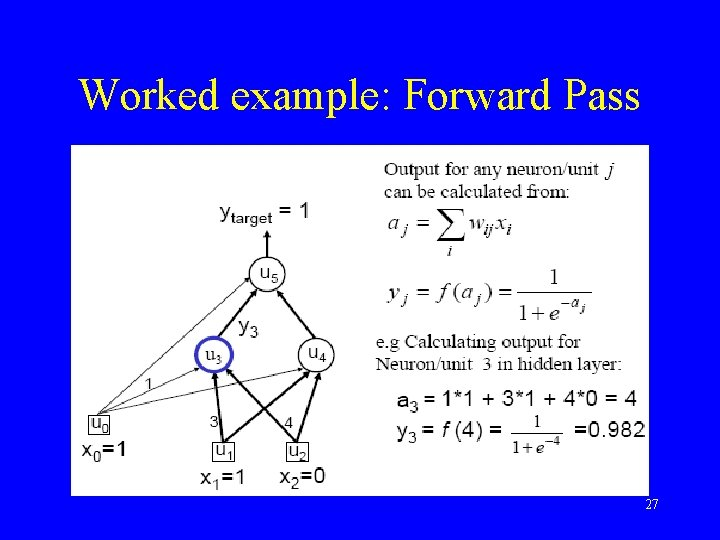Worked example: Forward Pass 27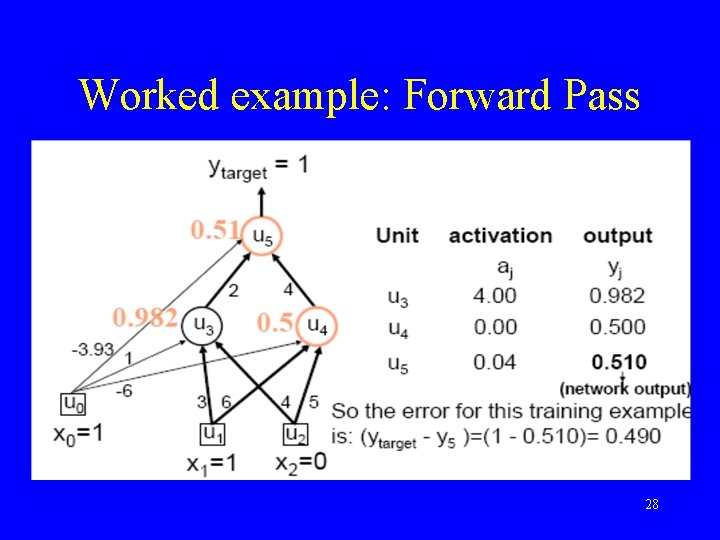Worked example: Forward Pass 28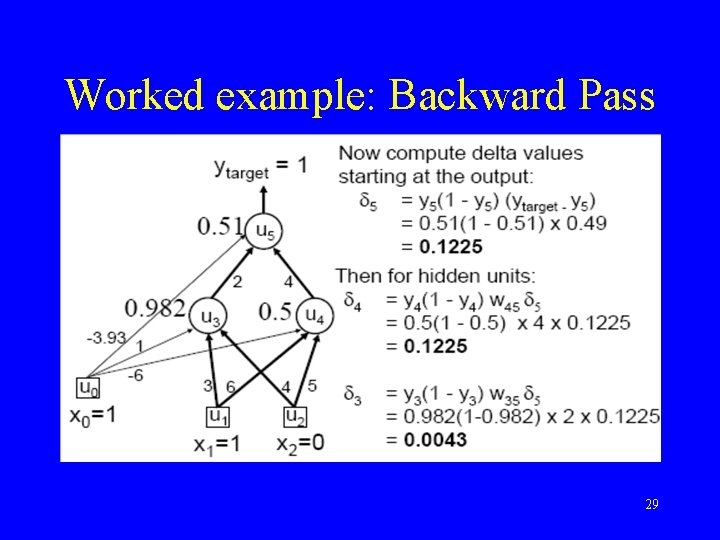Worked example: Backward Pass 29Worked example: Update Weights Using Generalized Delta Rule (BP) 30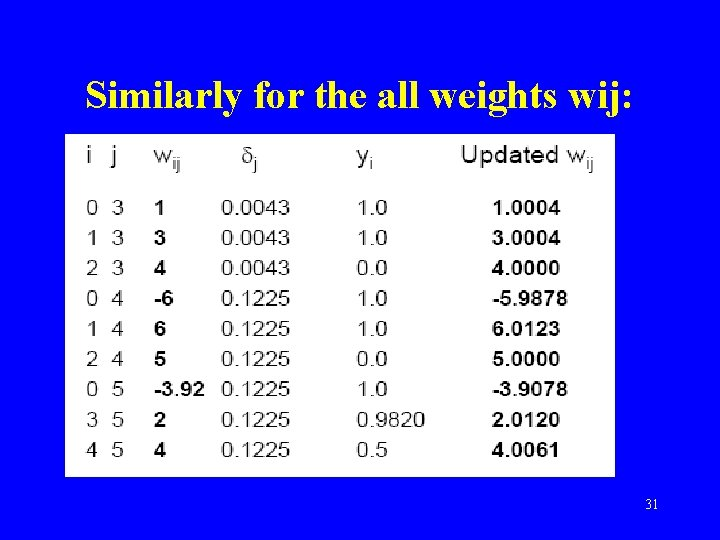Similarly for the all weights wij: 31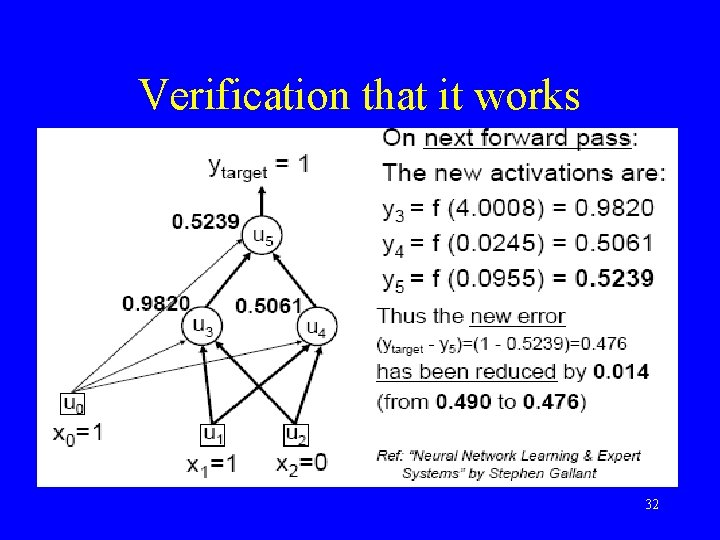Verification that it works 32Training • This was a single iteration of back-prop • Training requires many iterations with many training examples or epochs (one epoch is entire presentation of complete training set) • It can be slow ! • Note that computation in MLP is local (with respect to each neuron) • Parallel computation implementation is also possible 33Training and testing data • How many examples ? – The more the merrier ! • Disjoint training and testing data sets – learn from training data but evaluate performance (generalization ability) on unseen test data • Aim: minimize error on test data 34• Binary Logic Unit in an example – http: //www. cs. usyd. edu. au/~irena/ai 01/nn/5. ht ml • Multi. Layer Perceptron Learning Algorithm – http: //www. cs. usyd. edu. au/~irena/ai 01/nn/8. ht ml 35